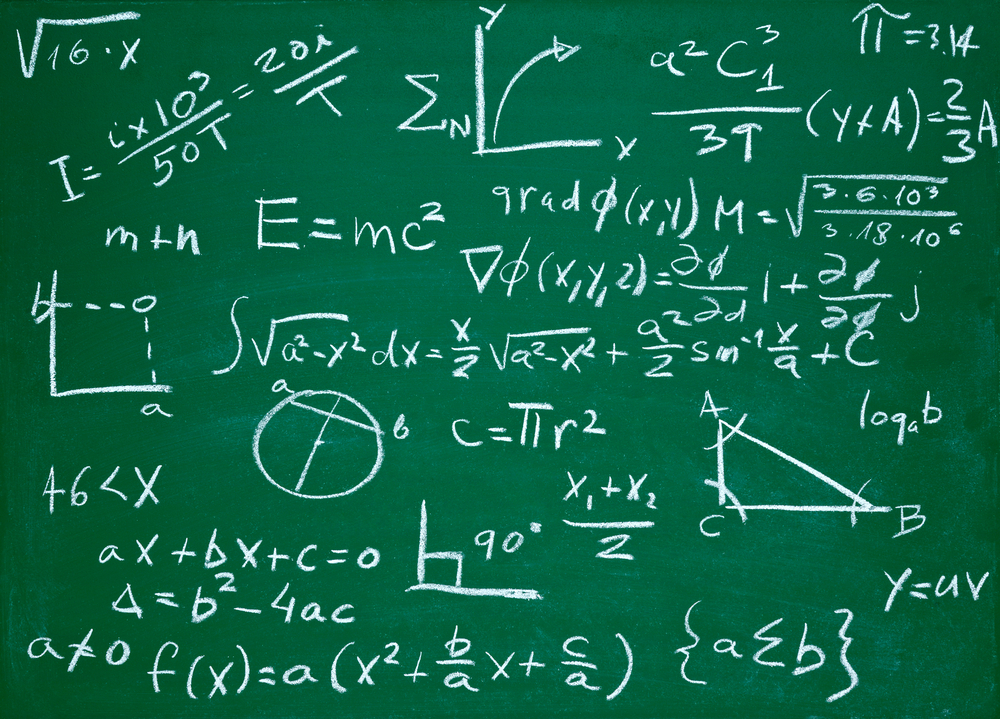Why did the negative number be hit with another negative number that gives a positive result?

in post •  last year

Well, the beginning of the calculation here is always from the zero point on the imaginary horizontal axis representing the infinity and zero in the middle (one side is positive and the other negative)

It is necessary to understand these two points:
The signal of a number in the equation (positive / negative) indicates the direction of our face as we walk.

The other signal is the direction of our steps (backward or forward, with the direction of our face as the first number sign)Annotated examples:
2 X 3 = 6

Three steps, two times.

We pointed towards the positive section of the axle, three steps forward two times.

2 X-3 = -6

Three steps, two times.

We directed towards the positive section of the axis, three steps back twice (up to 6-).

-2 X-3 = 6

Three steps, two times.

We turned to the negative section of the axis (because it is 2), three steps back twice (we reach 6 positive).